Next: Graphical solution of the Up: Graphical solution of 2-var Previous: The solution space of

#### Representing the Objective Function in the LP solution space

The most typical way to represent a two-variable function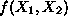is to perceive it as a surface in an (orthogonal) three-dimensional space, where two of the dimensions correspond to the independent variables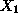and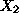, while the third dimension provides the function value for any pair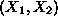. However, in the context of our discussion, we are interested in expressing the information contained in the two-var LP objective functionin the Cartesian plane defined by the two independent variablesand. For this purpose, we shall use the concept of contour plots. Contour plots depict a function by identifying the set of pointsthat correspond to a constant value of the function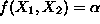, for any given range of's. The plot obtained for any fixed value ofis a contour of the function. Studying the structure of a contour is expected to identify some patterns that essentially depict some useful properties of the function.

In the case of LP's, the linearity of the objective function implies that any contour of it will be of the type: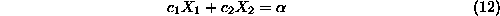i.e., a straight line. For a maximization (minimization) problem, this line will be called an isoprofit (isocost) line. Assuming that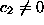(o.w., work with), Equation 12 can be rewritten as: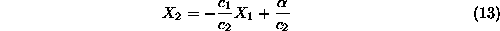which implies that by changing the value of, the resulting isoprofit/isocost lines have constant slope and varying intercept, i.e, they are parallel to each other (which makes sense, since by the definition of this cocnept, isoprofit/isocost lines cannot intersect). Hence, if we continously increasefrom some initial value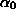, the corresponding isoprofit lines can be obtained by ``sliding'' the isprofit line corresponding to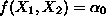parallel to itself, in the direction of increasing or decreasing intercepts, depending on whether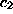is positive or negative.Next: Graphical solution of the Up: Graphical solution of 2-var Previous: The solution space of

UAL Data
Fri Jun 20 15:03:05 CDT 1997